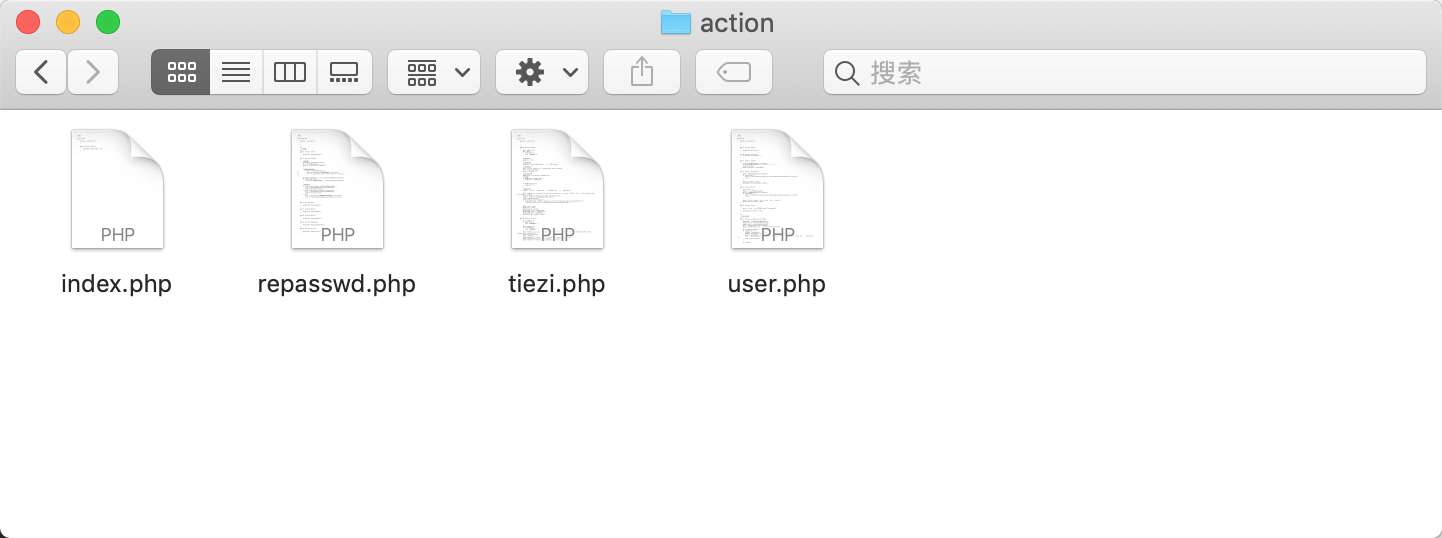1.找出关键位置

2.正向审计

3.反向审计

## 三、找出关键位置

### 3.1 找出控制器

``http://permeate.songboy.net/home/index.php ``

``http://permeate.songboy.net/home/index.php?m=tiezi&a=index&bk=6 ``

``````<?php require_once "../core/common.php"; include "./public/header.php";
includeAction("\$model","\$action"); include "./public/footer.php"; ``````

``````function includeAction(\$model, \$action) { //判断控制器是否存在 \$filePath = "./action/\$model.php"; if (is_readable(\$filePath)) { require_once \$filePath;
\$class = new \$model; if (is_callable(array(\$class, \$action))) {
\$class->\$action(); return true;
}
} //如果没有找到对应的控制器，直接调用模板文件 \$tplFilePath = "./tpl/\$model/\$action.php"; if (is_readable(\$tplFilePath)) { require_once \$tplFilePath; return true;
} echo '控制器或模板文件' . \$filePath . '不存在!'; die;
} ````````http://permeate.songboy.net/home/index.php?m=tiezi&a=index&bk=6 ``

``````<?php class tiezi { function __construct() {

} public function index() {
.....
\$data['count'] = \$count;
\$data['page_size'] = \$page_size;
\$data['page_count'] = \$page_count;
\$data['page_num'] = \$page_num;
displayTpl('tiezi/index', \$data);
} ``````

### 3.2 找出模板

``````/**
* 加载模板文件
* @param \$tplPath
*/ function displayTpl(\$tplPath, \$data = []) {
\$filePath = "./tpl/\$tplPath.php"; if (!is_readable(\$filePath)) { echo '模板文件' . \$filePath . '不存在!'; die;
} foreach (\$data as \$key => \$val) {
\$\$key = \$val;
} require_once \$filePath;

} ``````

### 3.3 验证位置

``public function index() { echo '11111111111'; ``

``222222222222222 <?php \$get = \$_GET; ?> <section class="section"> ``

## 四、正向审计

### 4.1 接收参数位置

`````` public function index() {
\$id = \$_GET['bk'];
\$bk = &\$id; //开始分页大小 \$page_size = 15; //获取当前页码 \$page_num = empty(\$_GET['page']) ? 1 : \$_GET['page']; //中间代码.................省略 \$data['bk'] = \$bk;
\$data['count'] = \$count;
\$data['page_size'] = \$page_size;
\$data['page_count'] = \$page_count;
\$data['page_num'] = \$page_num;
displayTpl('tiezi/index', \$data);
} ``````

### 4.2 模板位置是否过滤

``<div class="post-list-controller"> <div style="float: right"> <a class="btn btn-primary" href="fatie.php?bk=<?php echo \$bk ?>">发帖</a> </div> ``

### 4.3 漏洞验证

``http://permeate.songboy.net/home/index.php?m=tiezi&a=index&bk=6%22%3E%3Cscript%3Ealert(123)%3C/script%3E ``

## 五、反向审计

### 5.1 找出模板中的变量

``echo \\$([a-z]*) ``

### 5.2 查找变量来源

``````<?php include "public/header.php"; include "../core/common.php";

\$keywords = \$_REQUEST['keywords']; if (!empty(\$keywords)) {
\$where = " where title like '%\$keywords%' "; ``````

### 5.3 漏洞验证

``http://permeate.songboy.net/home/search.php?keywords=%E6%B5%8B%E8%AF%95%3Cscript%3Ealert(123)%3C/script%3E ``

P.S. 如果对笔者的Web安全文章较为感兴趣，可以关注笔者更多文章内容.

*本文作者：我是daxia，转自 FreeBuf